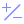#Random (PQL)

Returns a random integer between the lower and upper bounds provided.

• Returned Output: Numeric
• Library: PQL \ Common \ Math
• Version: 2018.00.000
• Compatibility:
• Can be combined with and other PQL function throughout the application.
• It CANNOT be used with MDX or VBA functions. But it can be used on MDX-based content in other parts of the application.

#### Syntax

Random( <Numeric> , <Numeric> )

* Click on the function's arguments above for more details on the input values.

• The first numeric argument is the lower bound; the second is the upper bound.
• The two values should be integers
##### Different Function types
• This function is like the Excel random function.
• Also see RandomDouble for random decimal numbers.

#### Examples

This example randomly returns "5" , picking a number between 0 and 10.

Random(0,10)

This example randomly returns -73 , picking a number between -100 and 0.

Random(-100,0)